## Properties of Proportions

The four properties that follow are not difficult to justify algebraically, but the details will not be presented here.

Property 1 (Means‐Extremes Property, or Cross‐Products Property): If a/b = c/d, then ad =bcConversely, if ad = bc ≠ 0, then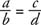and.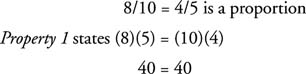Example 1: Find a if a/12 = 3/4.

By Property 1:Example 2: Is 3 : 4 = 7 : 8 a proportion?

No. If this were a proportion, Property 1 would produceProperty 2 (Means or Extremes Switching Property): If ab = cd and is a proportion, then both db = ca and ac = bd are proportions.

Example 3: 8/10 = 4/5 is a proportion. Property 2 says that if you were to switch the 8 and 5 or switch the 4 and 10, then the new statement is still a proportion.

If 8/10 = 4/5, then 5/10 = 4/8, or if 8/10 = 4/5, then 8/4 = 10/5.

Example 4: If x/5 = y/4, find the ratio of xy.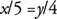Use the switching property of proportions and switch the means positions, the 5 and the y.Property 3 (Upside‐Down Property): If ab = cd, then ba = dc.

Example 5: If 9 a = 5 b ≠ 0, find the ratio.

First, apply the converse of the Cross Products Property and obtain the following:Next, proceed in one of the following two ways:

• Apply Property 3 to 9/5 = ba:
• Turn each side upside‐down.
• 5/9 = a/b, or a/b = 5/9
• Apply Property 2 to 9/ b = 5/ a:
• Switch the 9 and the a.
• ab = 5/9

Property 4 (Denominator Addition/Subtraction Property): If ab = cd, then ( a + b)/ b = ( c + d)/ d or ( a − b)/ b = ( c − d)/ d.

Example 6: If 5/8 = xy, then 13/8 = ?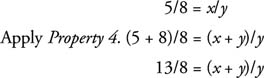Example 7: In Figure ABBC = 5/8. Find ACBC.Figure 1 Using the Segment Addition Postulate.

Recall that AB + BC = AC (Segment Addition Postulate).Example 8: A map is scaled so that 3 cm on the map is equal to 5 actual miles. If two cities on the map are 10 cm apart, what is the actual distance the cities are apart?

Let x = the actual distance.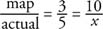Apply the Cross‐Products Property.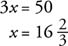The cities are 16⅔ miles apart.